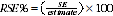4159.0.55.002 - General Social Survey: User Guide, Australia, 2010
ARCHIVED ISSUE Released at 11:30 AM (CANBERRA TIME) 07/12/2011
Page tools:Print All
Contents >> Data quality >> Sampling Error

SAMPLING ERROR

Sampling error is the difference between the published estimates, derived from a sample of persons, and the value that would have been produced if all persons in scope of the survey had been included. The magnitude of the sampling error associated with a sample estimate depends on the following factors:

• Sample design - there are many different methods which could have been used to obtain a sample from which to collect data. The final design attempted to make survey results as accurate as possible within cost and operational constraints. (Details of sample design are contained in Chapter 3: Survey Methodology).
• Sample size - the larger the sample on which the estimate is based, the smaller the associated sampling error.
• Population variability - the third factor which influences sampling error is the extent to which people differ on the particular characteristic being measured. This is referred to as the population variability for that characteristic. The smaller the population variability of a particular characteristic, the more likely it is that the population will be well represented by the sample, and therefore the smaller the sampling error. Conversely, the more variable the characteristic, the greater the sampling error.

Measures of sampling error

One measure of sampling variability is the Standard Error (SE) which indicates the extent to which an estimate might have varied by chance because only a sample of persons was included. There are approximately two chances in three that a sample estimate will differ by less than one standard error from the number that would have been obtained if all persons had been included in the survey, and about nineteen chances in twenty that the difference will be less than two standard errors.

Another measure of the likely difference is the Relative Standard Error (RSE), which is obtained by expressing the SE as a percentage of the estimate to which it relates:Very small estimates may be subject to such high RSEs so as to seriously detract from their value for most reasonable purposes. Only estimates with RSEs less than 25% are considered sufficiently reliable for most purposes. However, estimates with RSEs of 25% or more are included in all published 2010 GSS output. Estimates with an RSE of 25% to 50% are preceded by an asterisk (e.g. *3.4) to indicate that the estimate should be used with caution. Estimates with RSEs over 50% are indicated by a double asterisk (e.g.**0.6) and should be considered unreliable for most purposes.

RSEs for estimates from the 2010 GSS are available in 'actual' form, i.e. the RSE for each estimate produced can be calculated using the replicate weights. Replicate weighting is a process whereby a small group of persons or households in the sample are assigned a zero weight and then the remaining records are reweighted to the survey benchmark population. For the 2010 GSS this process was repeated 60 times to produce 60 replicate weights. These replicate weights are used for calculating the variances of the estimate for each replicate group and the original estimate, by squaring the difference and summing these differences over all of the 60 replicate groups. The difference between the replicate estimate and the original estimate is then used in calculating the standard error of the estimate.

 Previous Page Next Page